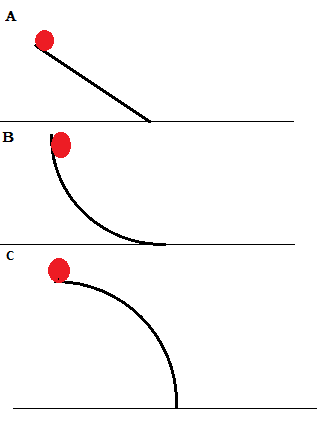# Describe the speed and acceleration of the ball released from the top of the track shown in the...

## Question:

Describe the speed and acceleration of the ball released from the top of the track shown in the figures below.## Force and Energy:

Force is the exertion of an effort on an object. Energy is the ability to exert the force. Energy and force are related, but energy is a scalar while force is a vector. Multiplying force by distance results in work, which is directly related to energy.

## Answer and Explanation:

The acceleration of the mass on ramp A will be constant due to the flat inclined surface. The acceleration of the mass on ramp B will initially be...

See full answer below.

Become a Study.com member to unlock this answer! Create your account